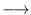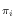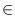Next: Singly Linked Lists ( Up: Basic Data Types Previous: Bounded Queues ( b_queue   Contents   Index

# Linear Lists ( list )

Definition

An instance L of the parameterized data type list<E> is a sequence of items (list<E>::item). Each item in L contains an element of data type E, called the element or value type of L. The number of items in L is called the length of L. If L has length zero it is called the empty list. In the sequel < x > is used to denote a list item containing the element x and L[i] is used to denote the contents of list item i in L.

#include < LEDA/core/list.h >

Types

 list::item the item type. list::value_type the value type.

Creation

 list L creates an instance L of type list and initializes it to the empty list.

Operations

Access Operations

 int L.length() returns the length of L. int L.size() returns L.length(). bool L.empty() returns true if L is empty, false otherwise. list_item L.first() returns the first item of L (nil if L is empty). list_item L.last() returns the last item of L. (nil if L is empty) list_item L.get_item(int i) returns the item at position i (the first position is 0). Precondition 0 < = i < L.length(). Note that this takes time linear in i. list_item L.succ(list_item it) returns the successor item of item it, nil if it = L.last(). Precondition it is an item in L. list_item L.pred(list_item it) returns the predecessor item of item it, nil if it = L.first(). Precondition it is an item in L. list_item L.cyclic_succ(list_item it) returns the cyclic successor of item it, i.e., L.first() if it = L.last(), L.succ(it) otherwise. list_item L.cyclic_pred(list_item it) returns the cyclic predecessor of item it, i.e, L.last() if it = L.first(), L.pred(it) otherwise. const E& L.contents(list_item it) returns the contents L[it] of item it. Precondition it is an item in L. const E& L.inf(list_item it) returns L.contents(it). const E& L.front() returns the first element of L, i.e. the contents of L.first(). Precondition L is not empty. const E& L.head() same as L.front(). const E& L.back() returns the last element of L, i.e. the contents of L.last(). Precondition L is not empty. const E& L.tail() same as L.back(). int L.rank(const E& x) returns the rank of x in L, i.e. its first position in L as an integer from [1...| L|] (0 if x is not in L). Note that this takes time linear in rank(x). Precondition operator== has to be defined for type E.

Update Operations

 list_item L.push(const E& x) adds a new item < x > at the front of L and returns it (L.insert(x,L.first(),leda::before)). list_item L.push_front(const E& x) same as L.push(x). list_item L.append(const E& x) appends a new item < x > to L and returns it (L.insert(x,L.last(),leda::behind)). list_item L.push_back(const E& x) same as L.append(x). list_item L.insert(const E& x, list_item pos, int dir=leda::behind) inserts a new item < x > behind (if dir=leda::behind) or in front of (if dir=leda::before) item pos into L and returns it (here leda::behind and leda::before are predefined constants). Precondition it is an item in L. E L.pop() deletes the first item from L and returns its contents. Precondition L is not empty. E L.pop_front() same as L.pop(). E L.pop_back() deletes the last item from L and returns its contents. Precondition L is not empty. E L.Pop() same as L.pop_back(). E L.del_item(list_item it) deletes the item it from L and returns its contents L[it]. Precondition it is an item in L. E L.del(list_item it) same as L.del_item(it). void L.erase(list_item it) deletes the item it from L. Precondition it is an item in L. void L.remove(const E& x) removes all items with contents x from L. Precondition operator== has to be defined for type E. void L.move_to_front(list_item it) moves it to the front end of L. void L.move_to_rear(list_item it) moves it to the rear end of L. void L.move_to_back(list_item it) same as L.move_to_rear(it). void L.assign(list_item it, const E& x) makes x the contents of item it. Precondition it is an item in L. void L.conc(list& L1, int dir = leda::behind) appends ( dir = leda : : behind or prepends (dir = leda::before) list L1 to list L and makes L1 the empty list. Precondition: L! = L1 void L.swap(list& L1) swaps lists of items of L and L1; void L.split(list_item it, list& L1, list& L2) splits L at item it into lists L1 and L2. More precisely, if it! = nil and L = x1,..., xk-1, it, xk+1,..., xn then L1 = x1,..., xk-1 and L2 = it, xk+1,..., xn. If it = nil then L1 is made empty and L2 a copy of L. Finally L is made empty if it is not identical to L1 or L2. Precondition it is an item of L or nil. void L.split(list_item it, list& L1, list& L2, int dir) splits L at item it into lists L1 and L2. Item it becomes the first item of L2 if dir==leda::before and the last item of L1 if dir=leda::behind. Precondition it is an item of L. void L.extract(list_item it1, list_item it2, list& L1, bool inclusive = true) extracts a sublist L1 from L. More precisely, if L = x1,..., xp, it1,..., it2, xs,..., xn then L1 = it1,..., it2 and L = x1,..., xp, xs,..., xn. (If inclusive is false then it1 and it2 remain in L.) Precondition it1 and it2 are items of L or nil. void L.apply(void (*f)(E& x)) for all items < x > in L function f is called with argument x (passed by reference). void L.reverse_items() reverses the sequence of items of L. void L.reverse_items(list_item it1, list_item it2) reverses the sub-sequence it1,..., it2 of items of L. Precondition it1 = it2 or it1 appears before it2 in L. void L.reverse() reverses the sequence of entries of L. void L.reverse(list_item it1, list_item it2) reverses the sequence of entries L[it1]...L[it2]. Precondition it1 = it2 or it1 appears before it2 in L. void L.permute() randomly permutes the items of L. void L.permute(list_item* I) permutes the items of L into the same order as stored in the array I. void L.clear() makes L the empty list.

Sorting and Searching

 void L.sort(int (*cmp)(const E& , const E& )) sorts the items of L using the ordering defined by the compare function cmp : E x Eint, withMore precisely, if (in1,..., inn) and (out1,..., outn) denote the values of L before and after the call of sort, then cmp(L[outj], L[outj+1]) < = 0 for 1 < = j < n and there is a permutationof [1..n] such that outi = infor 1 < = i < = n. void L.sort() sorts the items of L using the default ordering of type E, i.e., the linear order defined by function int compare (const E&, const E&). If E is a user-defined type, you have to provide the compare function (see Section User Defined Parameter Types). void L.merge_sort(int (*cmp)(const E& , const E& )) sorts the items of L using merge sort and the ordering defined by cmp. The sort is stable, i.e., if x = y and < x > is before < y > in L then < x > is before < y > after the sort. L.merge_sort() is more efficient than L.sort() if L contains large pre-sorted intervals. void L.merge_sort() as above, but uses the default ordering of type E. If E is a user-defined type, you have to provide the compare function (see Section User Defined Parameter Types). void L.bucket_sort(int i, int j, int (*b)(const E& )) sorts the items of L using bucket sort, where b maps every element x of L to a bucket b(x)[i..j]. If b(x) < b(y) then < x > appears before < y > after the sort. If b(x) = b(y), the relative order of x and y before the sort is retained, thus the sort is stable. void L.bucket_sort(int (*b)(const E& )) sorts list into increasing order as prescribed by b Precondition b is an integer-valued function on E. () merges the items of L and L1 using the ordering defined by cmp. The result is assigned to L and L1 is made empty. Precondition L and L1 are sorted incresingly according to the linear order defined by cmp. void L.merge(list& L1) merges the items of L and L1 using the default linear order of type E. If E is a user-defined type, you have to define the linear order by providing the compare function (see Section User Defined Parameter Types). void L.unique(int (*cmp)(const E& , const E& )) removes duplicates from L. Precondition L is sorted incresingly according to the ordering defined by cmp. void L.unique() removes duplicates from L. Precondition L is sorted increasingly according to the default ordering of type E and operator== is defined for E. If E is a user-defined type, you have to define the linear order by providing the compare function (see Section User Defined Parameter Types). list_item L.search(const E& x) returns the first item of L that contains x, nil if x is not an element of L. Precondition operator== has to be defined for type E. list_item L.min(const leda_cmp_base& cmp) returns the item with the minimal contents with respect to the linear order defined by compare function cmp. list_item L.min() returns the item with the minimal contents with respect to the default linear order of type E. list_item L.max(const leda_cmp_base& cmp) returns the item with the maximal contents with respect to the linear order defined by compare function cmp. list_item L.max() returns the item with the maximal contents with respect to the default linear order of type E.

Input and Output

Operators

 list& L = const list& L1 The assignment operator makes L a copy of list L1. More precisely if L1 is the sequence of items x1, x2,..., xn then L is made a sequence of items y1, y2,..., yn with L[yi] = L1[xi] for 1 < = i < = n. E& L[list_item it] returns a reference to the contents of it. list_item L[int i] an abbreviation for L.get_item(i). list_item L += const E& x same as L.append(x); returns the new item. ostream& ostream& out « const list& L same as L.print(out); returns out. istream& istream& in » list& L same as L.read(in)); returns in.

Iteration

forall_items(it, L) { the items of L are successively assigned to it'' }

forall(x, L) { the elements of L are successively assigned to x'' }

STL compatible iterators are provided when compiled with -DLEDA_STL_ITERATORS (see LEDAROOT/demo/stl/list.c for an example).

Implementation

The data type list is realized by doubly linked linear lists. Let c be the time complexity of the compare function and let d be the time needed to copy an object of type list<E>. All operations take constant time except of the following operations: search, revers_items, permute and rank take linear time O(n), item(i) takes time O(i), min, max, and unique take time O(c*n), merge takes time O(c*(n1 + n2)), operator=, apply, reverse, read, and print take time O(d*n), sort and merge_sort take time O(n*c*log n), and bucket_sort takes time O(e*n + j - i), where e is the time complexity of f. n is always the current length of the list.Next: Singly Linked Lists ( Up: Basic Data Types Previous: Bounded Queues ( b_queue   Contents   Index